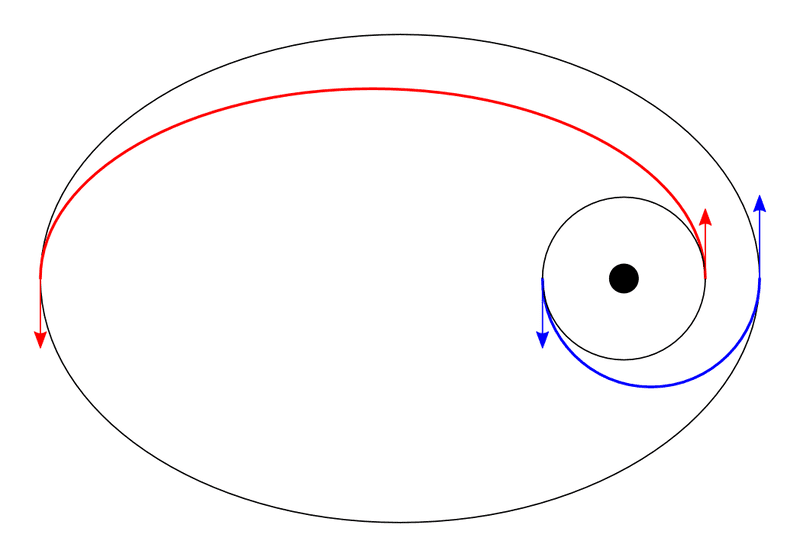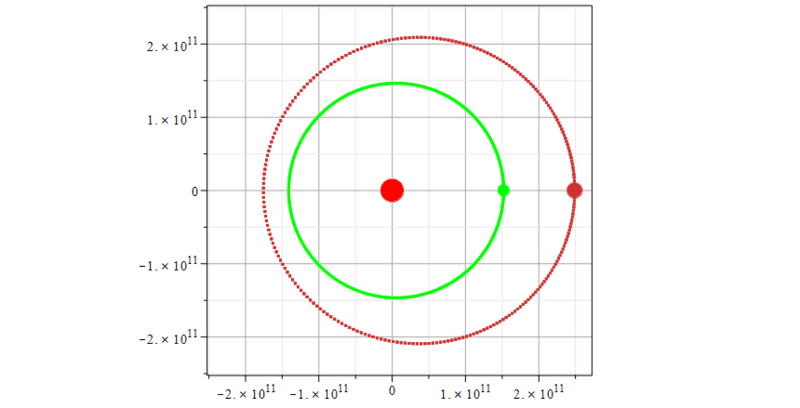# Computational Hohmann Transfer on MapleSoft

• Maple
• Lane Dillon

#### Lane Dillon

Hi,

I am trying to recreate an Interplanetary Hohmann transfer orbit from Earth to Mars similar to image A below (the red line). However, Earths orbit is not circular, It is more of an elliptical orbit rather than circular. I was able to successfully plot the orbits of both Earth and Mars correctly using the Verlet Algorithm (Image B), but I am having trouble with the Hohmann transfer. So far I've been trying to use the following equation but have not had any luck:

ΔV = sqrt(2*mu(sun)) * sqrt( R2 / (R1 * (R1+R2) ) )
R1 = radius of Earth orbit around sun (Aphelion)
R2 = radius of Mars orbit around sun (Aphelion)

Does anyone have any other possible equations to describe an interplanetary Hohamnn transfer? How would I use the above equation as a function of both orbits (Mars and Earth)? How could I split the above equation into X and Y components?

Thanks for the help!

Image A:Image B:#### Attachments

Why the equation doesn't work ?